## Getting Started with Mask R-CNN for Instance Segmentation

Instance segmentation is an enhanced type of object detection that generates a segmentation map for each detected instance of an object. Instance segmentation treats individual objects as distinct entities, regardless of the class of the objects. In contrast, semantic segmentation considers all objects of the same class as belonging to a single entity.

Mask R-CNN is a popular deep learning instance segmentation technique that performs pixel-level segmentation on detected objects . The Mask R-CNN algorithm can accommodate multiple classes and overlapping objects.

You can create a pretrained Mask R-CNN network using the `maskrcnn` object. The network is trained on the MS-COCO data set and can detect objects of 80 different classes. To perform instance segmentation, pass the pretrained network to the `segmentObjects` function.

If you want to modify the network to detect additional classes, or to adjust other parameters of the network, then you can perform transfer learning. For an example that shows how to train a Mask R-CNN, see Perform Instance Segmentation Using Mask R-CNN.

The Mask R-CNN network consists of two stages. The first stage is a region proposal network (RPN), which predicts object proposal bounding boxes based on anchor boxes. The second stage is an R-CNN detector that refines these proposals, classifies them, and computes the pixel-level segmentation for these proposals.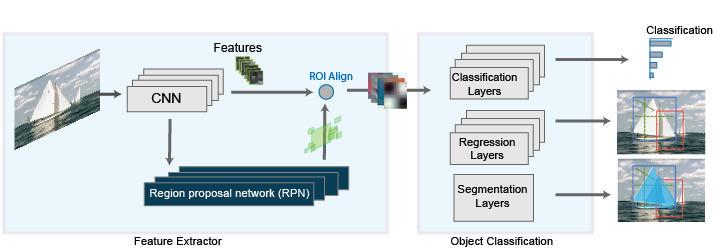The Mask R-CNN model builds on the Faster R-CNN model. Mask R-CNN replaces the ROI max pooling layer in Faster R-CNN with an `roiAlignLayer` that provides more accurate sub-pixel level ROI pooling. The Mask R-CNN network also adds a mask branch for pixel level object segmentation. For more information about the Faster R-CNN network, see Getting Started with R-CNN, Fast R-CNN, and Faster R-CNN.

This diagram shows a modified Faster R-CNN network on the left and a mask branch on the right.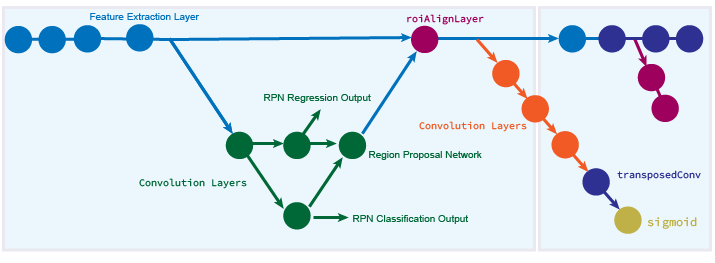To configure a Mask R-CNN network for transfer learning, specify the class names and anchor boxes when you create a `maskrcnn` object. You can optionally specify additional network properties including the network input size and the ROI pooling sizes.

### Prepare Mask R-CNN Training Data

To train a Mask R-CNN, you need the following data.

RGB image

RGB images that serve as network inputs, specified as H-by-W-by-3 numeric arrays.

For example, this sample RGB image is a modified image from the CamVid data set  that has been edited to remove personally identifiable information.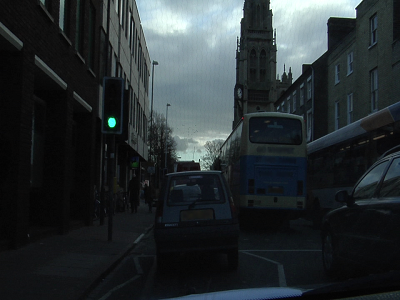Ground-truth bounding boxes

Bounding boxes for objects in the RGB images, specified as a NumObjects-by-4 matrix, with rows in the format [x y w h]).

For example, the `bboxes` variable shows the bounding boxes of six objects in the sample RGB image.

```bboxes = 394 442 36 101 436 457 32 88 619 293 209 281 460 441 210 234 862 375 190 314 816 271 235 305```

Instance labels

Label of each instance, specified as a NumObjects-by-1 string vector or a NumObjects-by-1 cell array of character vectors.)

For example, the `labels` variable shows the labels of six objects in the sample RGB image.

```labels = 6×1 cell array {'Person' } {'Person' } {'Vehicle'} {'Vehicle'} {'Vehicle'} {'Vehicle'}```

• Binary masks, specified as a logical array of size H-by-W-by-NumObjects. Each mask is the segmentation of one instance in the image.

• Polygon coordinates, specified as a NumObjects-by-2 cell array. Each row of the array contains the (x,y) coordinates of a polygon along the boundary of one instance in the image.

The Mask R-CNN network requires binary masks, not polygon coordinates. To convert polygon coordinates to binary masks, use the `poly2mask` function. The `poly2mask` function sets pixels that are inside the polygon to `1` and sets pixels outside the polygon to `0`. This code shows how to convert polygon coordinates in the `masks_polygon` variable to binary masks of size h-by-w-by-numObjects.

```denseMasks = false([h,w,numObjects]); for i = 1:numObjects denseMasks(:,:,i) = poly2mask(masks_polygon{i}(:,1),masks_polygon{i}(:,2),h,w); end```

For example, this montage shows the binary masks of six objects in the sample RGB image.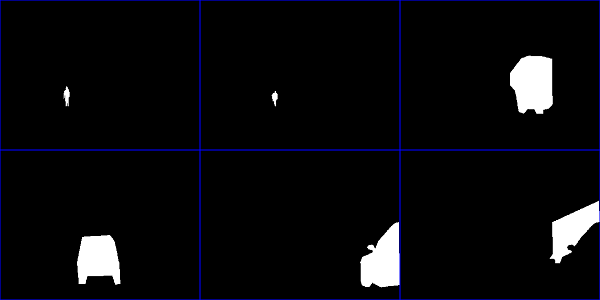#### Visualize Training Data

To display the instance masks over the image, use the `insertObjectMask`. You can specify a colormap so that each instance appears in a different color. This sample code shows how display the instance masks in the `masks` variable over the RGB image in the `im` variable using the `lines` colormap.

```imOverlay = insertObjectMask(im,masks,'Color',lines(numObjects)); imshow(imOverlay);```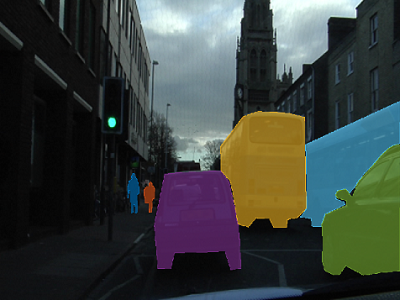To show the bounding boxes with labels over the image, use the `showShape` function. This sample code shows how to show labeled rectangular shapes with bounding box size and position data in the `bboxes` variable and label data in the `labels` variable.

```imshow(imOverlay) showShape("rectangle",bboxes,"Label",labels,"Color","red");```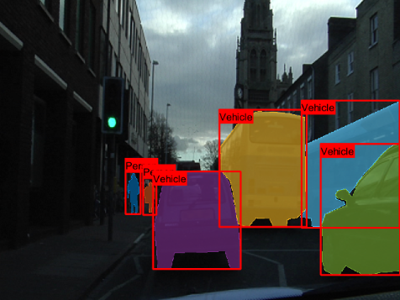Use a datastore to read data. The datastore must return data as a 1-by-4 cell array in the format {RGB images, bounding boxes, labels, masks}. The size of the images, bounding boxes, and masks must match the input size of the network. If you need to resize the data, then you can use the `imresize` to resize the RGB images and masks, and the `bboxresize` function to resize the bounding boxes.

#### Form Mini-Batches of Data

Training a Mask R-CNN network requires a custom training loop. To manage the mini-batching of observations in a custom training loop, create a `minibatchqueue` (Deep Learning Toolbox) object from the datastore. The `minibatchqueue` object casts data to a `dlarray` (Deep Learning Toolbox) object that enables auto differentiation in deep learning applications. If you have a supported GPU, then a `minibatchqueue` object also moves data to the GPU.

The `next` (Deep Learning Toolbox) function yields the next mini-batch of data from the `minibatchqueue`.

Train the model in a custom training loop. For each iteration:

• Read the data for current mini-batch using the `next` (Deep Learning Toolbox) function.

• Evaluate the model gradients using the `dlfeval` (Deep Learning Toolbox) function and a custom helper function that calculates the gradients and overall loss for batches of training data. You can calculate features of the training data and return the state information of the network using the `forward` function.

• Update the network learnable parameters using a function such as `adamupdate` (Deep Learning Toolbox) or `sgdmupdate` (Deep Learning Toolbox).

For an example that shows how to perform instance segmentation using a trained Mask R-CNN and how to set up a network for training, see Perform Instance Segmentation Using Mask R-CNN.

 He, Kaiming, Georgia Gkioxari, Piotr Dollár, and Ross Girshick. “Mask R-CNN.” ArXiv:1703.06870 [Cs], January 24, 2018. https://arxiv.org/pdf/1703.06870.

 Brostow, Gabriel J., Julien Fauqueur, and Roberto Cipolla. “Semantic Object Classes in Video: A High-Definition Ground Truth Database.” Pattern Recognition Letters 30, no. 2 (January 2009): 88–97. https://doi.org/10.1016/j.patrec.2008.04.005.